Factor Fun Factors and Prime Numbers Factor A

• Slides: 17Factor FunFactors and Prime Numbers • Factor: A whole number that divides another such number without a remainder. v 2 and 3 are factors of 6 v 4, 5, 10, etc. are factors of 120 • Prime numbers: any number that has exactly two factors. Namely, 1 and itself. v. Example: 2 = 1 X 2. v. Smallest Prime Numbers: 2, 3, 5, 7, 11, 13…… v. What is the next prime number? » 17Composite numbers and Factorization • Composite numbers: any number with a factor other than 1 and itself. v 1 is not Prime and is not a Composite! v 96 and 60 are composite numbers • Factorization means resolving or composing a number as a multiple of other numbers. v. Example: 96 = 32 X 3 = 4 X 8 X 3 = 2 X 2 X 2 X 3 v. Example: 60 = 2 X 30 = 2 X 15 =2 X 2 X 3 X 5 v. The last equality is the Prime Factorization • Where each factor is a prime numberFactors • 60 = 2 X 3 X 5. • So the factors of 60 are: Ø Ø Ø 1 2 3 2 X 2 5 2 X 3 2 X 5 2 X 2 X 3 3 X 5 2 X 2 X 5 2 X 3 X 5 2 X 2 X 3 X 5 = 1 = 2 = 3 = 4 = 5 = 6 = 10 = 12 = 15 = 20 = 30 = 60 How the Factors combine to make 60: 60 = 1 X 60 = 2 X 30 = 3 X 20 = 4 X 15 = 5 X 12 = 6 X 10 • The greatest factor of a number is the number itself.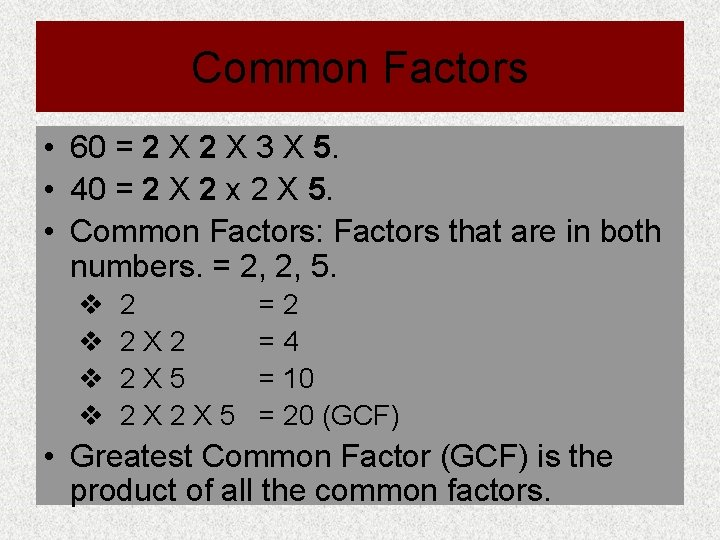Common Factors • 60 = 2 X 3 X 5. • 40 = 2 X 2 x 2 X 5. • Common Factors: Factors that are in both numbers. = 2, 2, 5. v v 2 2 X 5 2 X 2 X 5 =2 =4 = 10 = 20 (GCF) • Greatest Common Factor (GCF) is the product of all the common factors.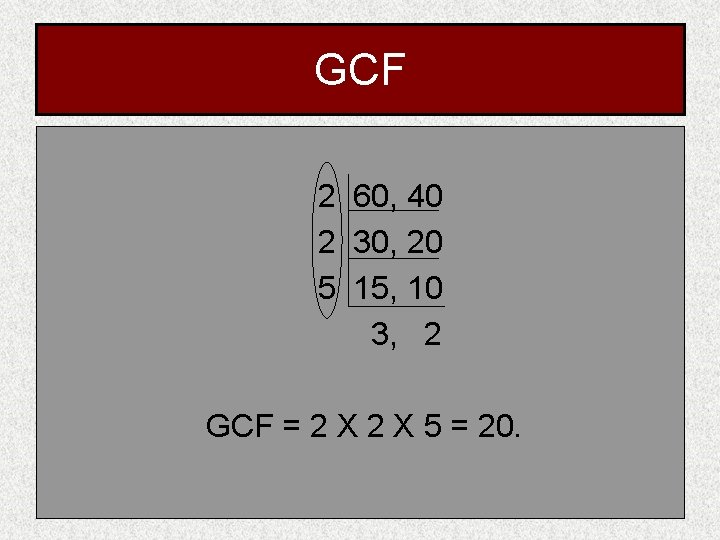GCF 2 60, 40 2 30, 20 5 15, 10 3, 2 GCF = 2 X 5 = 20.GCF • 60 =2 X 2 X 3 X 5 • 40 =2 X 2 X 2 X 5 • GCF = 2 X 5 = 20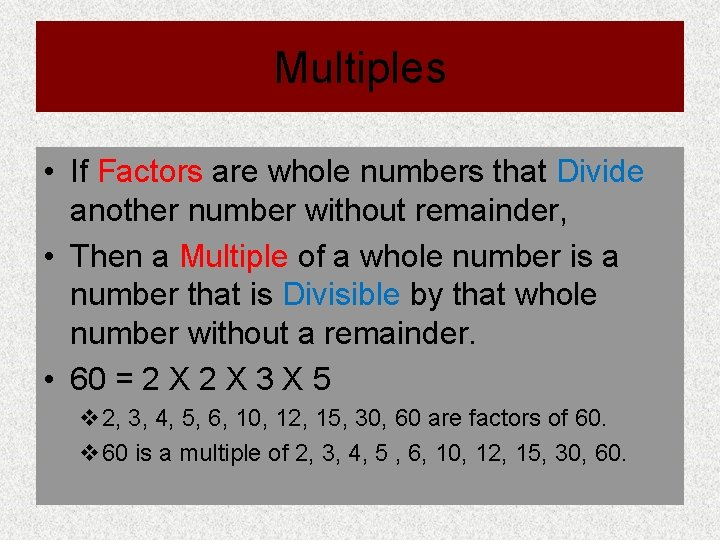Multiples • If Factors are whole numbers that Divide another number without remainder, • Then a Multiple of a whole number is a number that is Divisible by that whole number without a remainder. • 60 = 2 X 3 X 5 v 2, 3, 4, 5, 6, 10, 12, 15, 30, 60 are factors of 60. v 60 is a multiple of 2, 3, 4, 5 , 6, 10, 12, 15, 30, 60.LCM • Multiples of 60: v 60 X 1 = 60, 60 X 2 = 120 …… so on v 60, 120, 180, 240, 300, 360, 420, …. . v Lowest multiple of a number is the number itself • Multiples of 40: v 40 X 1 = 40, 40 X 2 = 80 …… so on v 40, 80, 120, 160, 200, 240, 280, 320, 360, 400, …. v Common Multiples are 120, 240, 360, ……. v Lowest Common Multiple (LCM) : the smallest number that is divisible by both numbers without a remainder = 120. v There is no Greatest Common MultipleLCM 2 60, 40 2 30, 20 5 15, 10 3, 2 LCM = 2 X 5 X 3 X 2 = 120.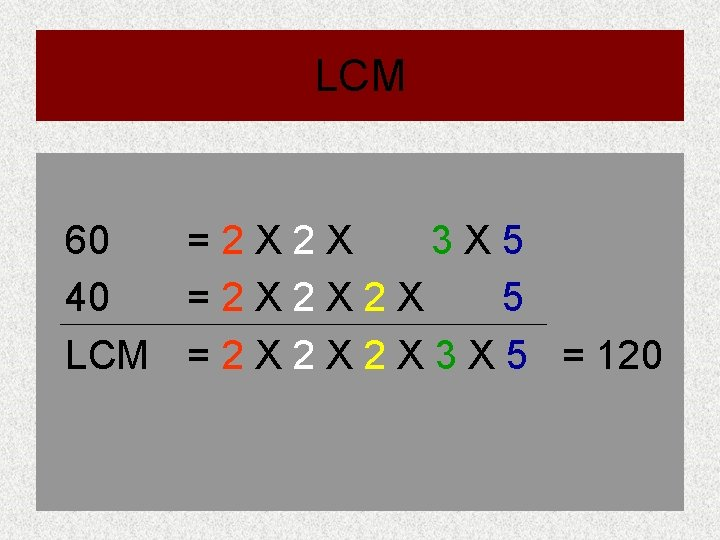LCM 60 =2 X 2 X 3 X 5 40 =2 X 2 X 2 X 5 LCM = 2 X 2 X 3 X 5 = 120GCF and LCM • 60 • 40 • GCF • 60 • 40 • LCM =2 X 2 X 3 X 5 =2 X 2 X 2 X 5 = 20 =2 X 2 X 3 X 5 =2 X 2 X 2 X 3 X 5 = 120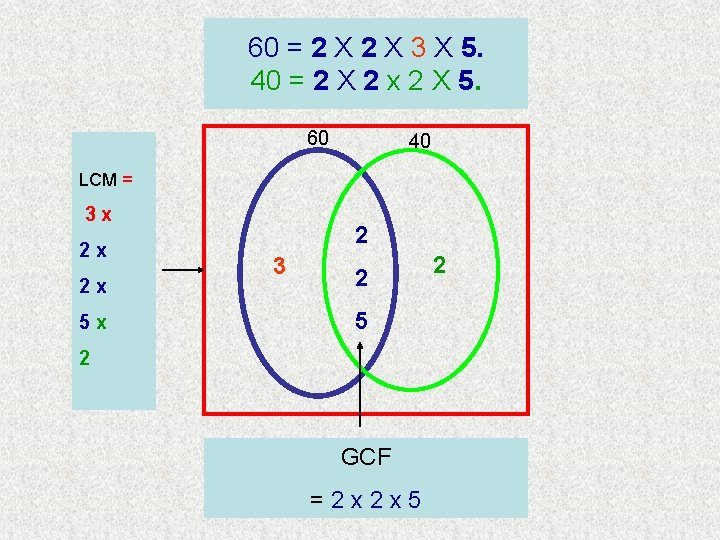60 = 2 X 3 X 5. 40 = 2 X 2 x 2 X 5. 60 40 LCM = 3 x 2 x 2 x 5 x 2 3 2 5 2 GCF =2 x 2 x 5 2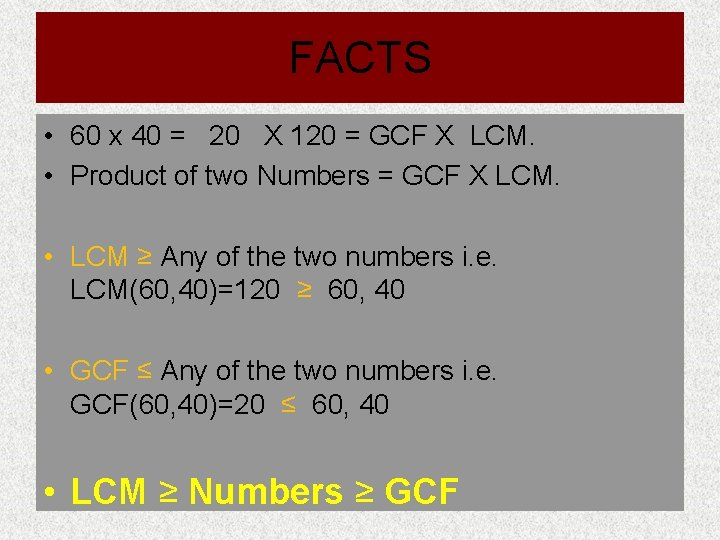FACTS • 60 x 40 = 20 X 120 = GCF X LCM. • Product of two Numbers = GCF X LCM. • LCM ≥ Any of the two numbers i. e. LCM(60, 40)=120 ≥ 60, 40 • GCF ≤ Any of the two numbers i. e. GCF(60, 40)=20 ≤ 60, 40 • LCM ≥ Numbers ≥ GCFActivities • Visit any of the following websites for activity on GCF and LCM. • http: //schools. parkhill. k 12. mo. us/Congress/Team 7 A/Module%203%20 Activit ies%20 by%20 Mr. %20 Kalis. htm • http: //mason. gmu. edu/~mmankus/talks/whole/rods 001. htm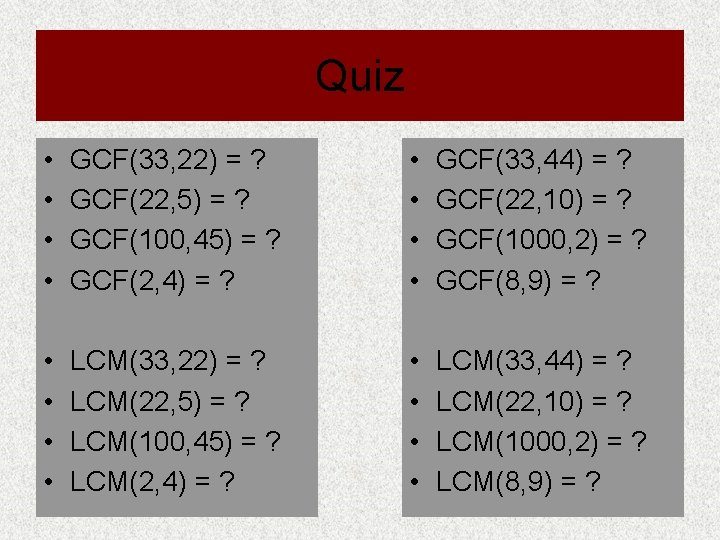Quiz • • GCF(33, 22) = ? GCF(22, 5) = ? GCF(100, 45) = ? GCF(2, 4) = ? • • GCF(33, 44) = ? GCF(22, 10) = ? GCF(1000, 2) = ? GCF(8, 9) = ? • • LCM(33, 22) = ? LCM(22, 5) = ? LCM(100, 45) = ? LCM(2, 4) = ? • • LCM(33, 44) = ? LCM(22, 10) = ? LCM(1000, 2) = ? LCM(8, 9) = ?Quiz Answers • • GCF(33, 22) = 11 GCF(22, 5) = 1 GCF(100, 45) = 5 GCF(2, 4) = 2 • • GCF(33, 44) = 11 GCF(22, 10) = 2 GCF(1000, 2) = 2 GCF(8, 9) = 1 • • LCM(33, 22) = 66 LCM(22, 5) = 110 LCM(100, 45) = 900 LCM(2, 4) = 4 • • LCM(33, 44) = 132 LCM(22, 10) = 110 LCM(1000, 2) = 1000 LCM(8, 9) = 72